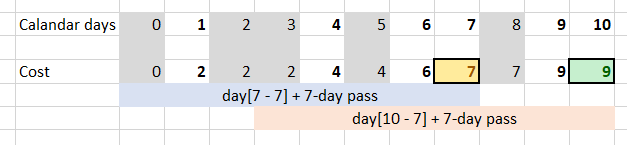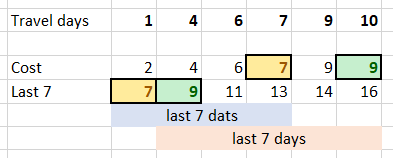## Sunday, January 27, 2019

### Dynamic Programming: Minimum Travel Cost

The higher is the bar, the more you're expected to use dynamic programming (DP) during an interview. This technique requires a lot of practice to grasp; if you've mastered the recursion, DP is the next level.
This problem appeared on LeetCode weekly contest #121, and it's a good problem to practice the DP thinking.

# Problem Description

In a country popular for train travel, you have planned some train travelling one year in advance. The days of the year that you will travel is given as an array days. Each day is an integer from 1 to 365.
Train tickets are sold in 3 different ways:
• 1-day pass is sold for costs dollars;
• 7-day pass is sold for costs dollars;
• 30-day pass is sold for costs dollars.
The passes allow that many days of consecutive travel. For example, if we get a 7-day pass on day 2, then we can travel for 7 days: day 2, 3, 4, 5, 6, 7, and 8.
Return the minimum number of dollars you need to travel every day in the given list of days.

# Coding Practice

Try solving this problem before moving on to the solutions. It is available on LeetCode Online Judge (983. Minimum Cost For Tickets). Also, as you read through a solution, try implementing it yourself.
LeetCode is my favorite destinations for algorithmic problems. It has 978 problems and counting, with test cases to validate the correctness, as well as computational and memory complexity. There is also a large community discussing different approaches, tips and tricks.

# Intuition

For each travel day, we can buy a one-day ticket, or use 7-day or 30-day passes as if we would have purchased it 7 or 30 days ago. We need to track rolling costs for at least 30 days back, and use them to pick the cheapest option for the next travel day.
Here, we can use two approaches: track cost for all calendar days, or process only travel days. The first approach is simpler to implement, but it's slower. Since the problem is limited to one calendar year, it does not make much of a difference; for a generalized problem I would recommend the second approach.

# 1. Track calendar days

We track the minimum cost for all calendar days in `dp`. For non-travel days, the cost stays the same as for the previous day. For travel days, it's a minimum of yesterday's cost plus single-day ticket, or cost for 8 days ago plus 7-day pass, or cost 31 days ago plus 30-day pass.``````int mincostTickets(vector<int>& days, vector<int>& costs) {
unordered_set<int> travel(begin(days), end(days));
int dp = {};
for (int i = 1; i < 366; ++i) {
if (travel.find(i) == travel.end()) dp[i] = dp[i - 1];
else dp[i] = min({ dp[i - 1] + costs, dp[max(0, i - 7)] + costs, dp[max(0, i - 30)] + costs});
}
return dp;
}
``````

# Memory Optimization

In the previous solution, we store cost for all calendar days. However, since we only look 31 days back, we can just store the cost for last 31 days in a rolling array.
``````int mincostTickets(vector<int>& days, vector<int>& costs) {
unordered_set<int> travel(begin(days), end(days));
int dp = {};
for (int i = 1; i < 366; ++i) {
if (travel.find(i) == travel.end()) dp[i % 31] = dp[(i - 1) % 31];
else dp[i % 31] = min({ dp[(i - 1) % 31] + costs,
dp[max(0, i - 7) % 31] + costs, dp[max(0, i - 30) % 31] + costs });
}
return dp[365 % 31];
}
``````

# Complexity analysis

• Time Complexity: O(N), where N is the number of calendar days.
• Space Complexity: O(N) or O(31) for the optimized solution. Stricter, it's a maximum duration among all pass types.

# 2. Track travel days

We track the minimum `cost` for each travel day. We process only travel days and store {day, cost} for 7-and 30-day passes in the `last7` and `last30` queues. After a pass 'expires', we remove it from the queue. This way, our queues only contains travel days for the last 7 and 30 days, and the cheapest pass prices are in the front of the queues.``````int mincostTickets(vector<int>& days, vector<int>& costs, int cost = 0) {
queue<pair<int, int>> last7, last30;
for (auto d : days) {
while (!last7.empty() && last7.front().first + 7 <= d) last7.pop();
while (!last30.empty() && last30.front().first + 30 <= d) last30.pop();
last7.push({ d, cost + costs });
last30.push({ d, cost + costs });
cost = min({ cost + costs, last7.front().second, last30.front().second });
}
return cost;
}
``````

# Complexity analysis

• Time Complexity: O(n), where n is the number of travel days.
• Space Complexity: O(38). Stricter, it's a sum of duration for all pass types (1 + 7 + 30 in our case).

1.Great explanation. Thanks

2.Golang

func mincostTickets(days []int, costs []int) int {
n := len(days)
dp := make([]int, n+1)
i7 := 1
i30 := 1
for i := 1; i <= n; i++ {
for ;i > i7 && days[i-1] - days[i7-1] + 1 > 7; i7++ {
}
for ;i > i30 && days[i-1] - days[i30-1] + 1 > 30; i30++ {
}
dp[i] = dp[i-1] + costs
dp[i] = min(dp[i], dp[i7-1] + costs)
dp[i] = min(dp[i], dp[i30-1] + costs)

}
return dp[n]
}

func min(a, b int) int {
if a < b {
return a
} else {
return b
}
}